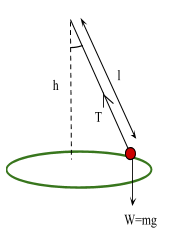# Motion In A Circle

Contents

## Summary

• So for an object moving in a circle, there is an inward force acting upon it in order to cause its inward acceleration. This is known as Centripetal Force.
• Angular velocity is basically the rate of change of angle when an object is in a circular motion:$\omega \quad =\quad 2\pi f\quad { rad }/{ sec }$.  Where f is the frequency of rotation and$\omega$  is the angular velocity.
• Magnitude of the acceleration${ a }_{ c }$  for uniform circular motion is${ a }_{ c }\quad =\quad { \omega }^{ 2 }r$.
• Centripetal force:$F\quad =\quad \frac { m{ v }^{ 2 } }{ r }$
• For the Conical Pendulum:
• the vertical component is:$Tcos\theta \quad =\quad mg$
• the horizontal component is:$Tsin\theta \quad =\quad m{ \omega }^{ 2 }r$

#### Centripetal Force

Any object that is moving in a circle e.g earth orbiting the sun, it continuously changes direction in result of a force acting on the object. This force is called ‘Centripetal force’.#### Angular Speed

Angular velocity is basically the rate of change of angle when an object is in a circular motion, commonly denoted ω (called omega) and measured in radians per second. It could also be measured in revolutions per second. Omega ω can be calculated using the formula:$\omega \quad =\quad 2\pi f\quad { rad }/{ sec }$

Where f is the frequency of rotation and$T\quad =\quad \frac { 1 }{ f }$  is the time period of a single revolution.

For circular motion at constant speed, the direction of the object is continuously changing this is because the velocity is always tangential to the circular path, and magnitude remains constant. Therefore, the object has an acceleration.

This relation can be shown through a formula as the magnitude of the acceleration${ a }_{ c }$  for uniform circular motion with speed v in a path of radius r is:${ a }_{ c }\quad =\quad \frac { { v }^{ 2 } }{ r }$

and that the direction of the acceleration is inward toward the center of the circular path.

To write it in terms of angular speed:

We know:$\omega \quad =\quad \frac { v }{ r }$

Therefore:${ a }_{ c }\quad =\quad { \omega }^{ 2 }r$

Newton’s second law requires that there be a net force on the object equal in magnitude to${ ma }_{ c }$  and in the direction of${ a }_{ c }$ . Hence:$F\quad =\quad m{ a }_{ c }$

Substituting the value of${ a }_{ c }$,  we get:$F\quad =\quad { m\omega }^{ 2 }r$  or$F\quad =\quad \frac { m{ v }^{ 2 } }{ r }$

#### Example #1

Q. An object that moves in uniform circular motion has a centripetal acceleration of$13\quad { m }/{ { s }^{ 2 } }$. If the radius of the motion is 0.02 m, what is the frequency of the motion?

Solution:${ a }_{ c }\quad =\quad \frac { { v }^{ 2 } }{ r }$$13\quad =\quad \frac { { v }^{ 2 } }{ 0.02 }$$v\quad =\quad 0.51\quad { m }/{ s }$

As:$\omega \quad =\quad 2\pi f$  and$\omega \quad =\quad \frac { v }{ r }$

Equating both equations:$2\pi f\quad =\quad \frac { v }{ r }$

As$f\quad =\quad \frac { 1 }{ T }$$v\quad =\quad \frac { 2\pi r }{ T }$$0.51\quad =\quad \frac { 6.28\quad (0.02) }{ T }$$T\quad =\quad 0.25\quad s$$f\quad =\quad \frac { 1 }{ 0.25 }$$f\quad =\quad 4\quad Hz$

#### The Conical Pendulum

A conical Pendulum consists of a bob of mass ‘m’ revolving in a horizontal circle with constant speed ‘v’ at the end of a string of length ‘l’. The string makes a constant angle$\theta$  with the vertical.Tension T in the string can be resolved into two components:

vertical component$Tcos\theta$  and horizontal component$Tsin\theta$.

The vertical component of the tension force balances the weight of the object.$Tcos\theta \quad =\quad mg\quad \quad \Rightarrow \quad \quad (1)$

Moving on to the horizontal component.

The bob attached to the string experiences a centripetal acceleration${ a }_{ c }\quad =\quad { \omega }^{ 2 }r$.

Hence, it is subject to a centripetal force$F\quad =\quad m{ a }_{ c }$  becomes$F\quad =\quad { m\omega }^{ 2 }r$.

This force is provided by the component of the string tension which acts towards the centre of the circle.$Tsin\theta \quad =\quad m{ \omega }^{ 2 }r\quad \quad \Rightarrow \quad \quad (2)$

Taking ratio of both equations (1) and (2), we get:$tan\theta \quad =\quad \frac { { \omega }^{ 2 }r }{ g }$

Since height:$h\quad =\quad lcos\theta$$tan\theta \quad =\quad \frac { r }{ h }$

Therefore:$\omega \quad =\quad \sqrt { \frac { g }{ h } }$